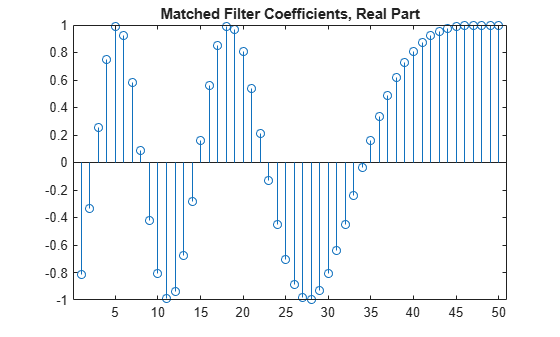# getMatchedFilter

System object: phased.LinearFMWaveform
Package: phased

Matched filter coefficients for waveform

## Syntax

```Coeff = getMatchedFilter(H) Coeff = getMatchedFilter(H,'FrequencyOffset',FOFFSET) ```

## Description

`Coeff = getMatchedFilter(H)` returns the matched filter coefficients for the linear FM waveform object `H`. `Coeff` is a column vector.

`Coeff = getMatchedFilter(H,'FrequencyOffset',FOFFSET)` adds a frequency offset when matched filter coefficients are generated. `FOFFSET` must be a scalar. This option is available when you set the `FrequencyOffsetSource` property to ```'Input port'``` for the input object, `H`.

## Examples

expand all

Get the matched filter coefficients for a linear FM pulse.

```waveform = phased.LinearFMWaveform('PulseWidth',5e-05,... 'SweepBandwidth',1e5,'OutputFormat','Pulses'); coeff = getMatchedFilter(waveform); stem(real(coeff)) title('Matched Filter Coefficients, Real Part')```